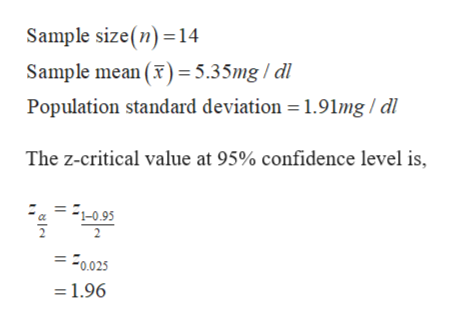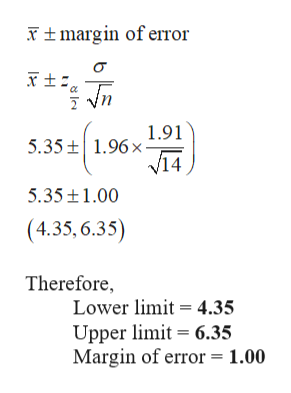# Overproduction of uric acid in the body can be an indication of cell breakdown. This may be an advance indication of illness such as gout, leukemia, or lymphoma.† Over a period of months, an adult male patient has taken fourteen blood tests for uric acid. The mean concentration was x = 5.35 mg/dl. The distribution of uric acid in healthy adult males can be assumed to be normal, with σ = 1.91 mg/dl.(a) Find a 95% confidence interval for the population mean concentration of uric acid in this patient's blood. What is the margin of error? (Round your answers to two decimal places.) lower limit     upper limit     margin of error     (b) What conditions are necessary for your calculations? (Select all that apply.)normal distribution of uric acidn is largeσ is unknownnσ is knownuniform distribution of uric acid(c) Interpret your results in the context of this problem.The probability that this interval contains the true average uric acid level for this patient is 0.95.There is not enough information to make an interpretation.    The probability that this interval contains the true average uric acid level for this patient is 0.05.There is a 5% chance that the confidence interval is one of the intervals containing the population average uric acid level for this patient.There is a 95% chance that the confidence interval is one of the intervals containing the population average uric acid level for this patient.(d) Find the sample size necessary for a 95% confidence level with maximal margin of error E = 1.16 for the mean concentration of uric acid in this patient's blood. (Round your answer up to the nearest whole number.)   blood tests

Question
2696 views

Overproduction of uric acid in the body can be an indication of cell breakdown. This may be an advance indication of illness such as gout, leukemia, or lymphoma.† Over a period of months, an adult male patient has taken fourteen blood tests for uric acid. The mean concentration was x = 5.35 mg/dl. The distribution of uric acid in healthy adult males can be assumed to be normal, with σ = 1.91 mg/dl.

(a) Find a 95% confidence interval for the population mean concentration of uric acid in this patient's blood. What is the margin of error? (Round your answers to two decimal places.)
 lower limit upper limit margin of error

(b) What conditions are necessary for your calculations? (Select all that apply.)
normal distribution of uric acid
n is large
σ is unknown
nσ is knownu
niform distribution of uric acid

(c) Interpret your results in the context of this problem.
The probability that this interval contains the true average uric acid level for this patient is 0.95.
There is not enough information to make an interpretation.
The probability that this interval contains the true average uric acid level for this patient is 0.05.
There is a 5% chance that the confidence interval is one of the intervals containing the population average uric acid level for this patient.
There is a 95% chance that the confidence interval is one of the intervals containing the population average uric acid level for this patient.

(d) Find the sample size necessary for a 95% confidence level with maximal margin of error E = 1.16 for the mean concentration of uric acid in this patient's blood. (Round your answer up to the nearest whole number.)

blood tests

check_circle

Step 1

The provided information are:help_outlineImage TranscriptioncloseSample size(n)14 Sample mean ()=5.35mg / dl Population standard deviation = 1.91mg/dl The z-critical value at 95% confidence level is = -10.95 = 0.025 1.96 fullscreen
Step 2

(a) The required 95% confidence interval is,help_outlineImage Transcriptionclosetmargin of eiror 1.91 5.35 1.96x VI4 5.35t1.00 (4.35,6.35) Therefore, Lower limit 4.35 Upper limit 6.35 Margin of error 1.00 바두 fullscreen
Step 3

(b) The required con...

### Want to see the full answer?

See Solution

#### Want to see this answer and more?

Solutions are written by subject experts who are available 24/7. Questions are typically answered within 1 hour.*

See Solution
*Response times may vary by subject and question.
Tagged in

### Hypothesis Testing